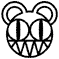# Proof (geometry)

A proof in mathematical terms is something that which can be proven using another proof.

## Formal Definition

Define the problematic set S such that all elements ai are unsolved problems. Therefore, the method ξ(ai) will return a solution that makes ai no longer an element in the problematic set.

## Famous Examples

1. Proof: All bedrooms will tend towards an overall state of disorganization with efficiency lim n→∞ Θ(ln(n)):
Define a bedroom Β0 as a teenage bedroom's initial state at time n = 0. Because of the Teenager Inefficiency Theorem,
Βi = (Βi-1 + Σ(ln(n)))/Βi-1, n = 0, 1, 2, ..., i
Therefore, disorganizedness Βi will tend to infinity, but at an increasedly slower rate, mimicking a teenage bedroom that, once it has reached a threshold messiness, it is difficult for it to increase the messiness.
So using the deMarconi Simplification Axiom by transposing Βi into an algorithmical efficiency expression, one gets that which was meant to be proven.

Q.E.D.This page has received the blessing of The Great Gozimnot of Mathematics, and is therefore mathematically correct in all aspects.